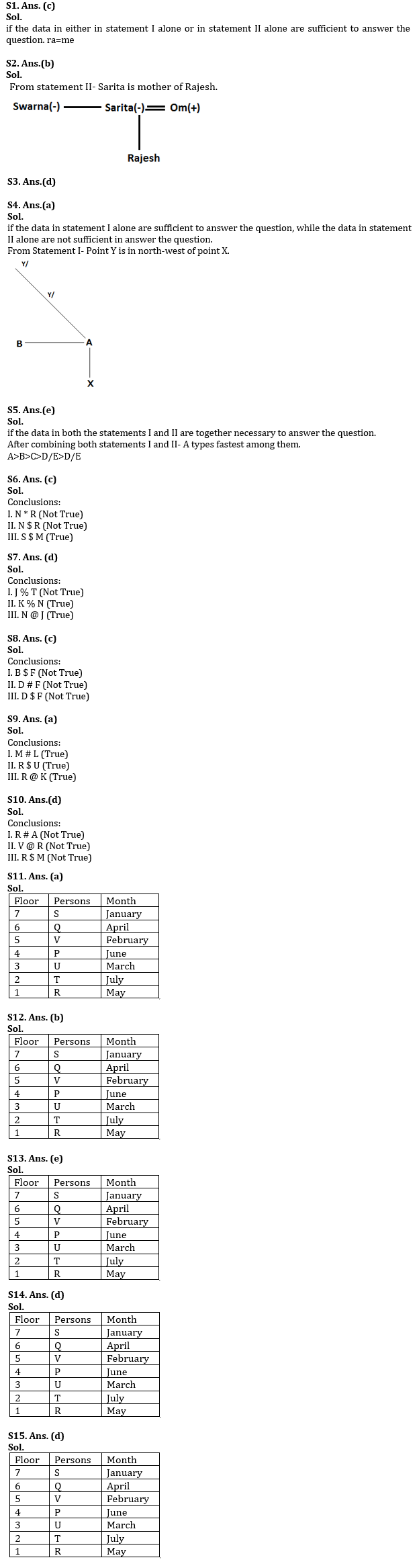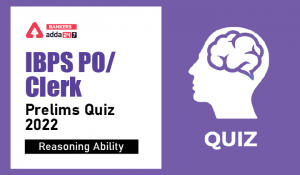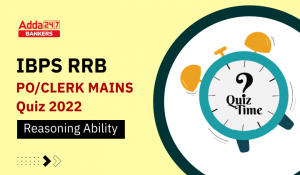Latest Banking jobs   »   Reasoning Ability Quiz For RBI Assistant/...

# Reasoning Ability Quiz For RBI Assistant/ ESIC UDC Mains 2022- 24th April

Directions (1-5): Each of the following questions below consists of a question and two statements numbered I and II given below it. You have to decide whether the data provided in the statements are sufficient to answer the question. Read both the statements and give answer.

Q1. What does ‘ra’ mean in the code language?
I. ‘st qm ra’ means ‘Look at me’ and ‘qm ms st rt’ means ‘don’t look at him’ in that code language.
II. ‘ka tv ne ra’ means ‘take me and go’ and ‘vw wx si ra’ means ‘either me or you’ in that code language.
(a) if the data in statement I alone are sufficient to answer the question, while the data in statement II alone are not sufficient in answer the question.
(b) if the data in statement II alone are sufficient to answer the question, while the data in statement I alone are not sufficient to answer the question.
(c) if the data in either in statement I alone or in statement II alone are sufficient to answer the question.
(d) if the data in both the statements I and II together are not sufficient to answer the question.
(e) if the data in both the statements I and II are together necessary to answer the question.

Q2. How is Sarita related to Rajesh?
I. Soni, the cousin of Rajesh, is the niece of Sarita.
II. Swarna is sister of Sarita who is the wife of Om. Om is the father of Rajesh.
(a) if the data in statement I alone are sufficient to answer the question, while the data in statement II alone are not sufficient in answer the question.
(b) if the data in statement II alone are sufficient to answer the question, while the data in statement I alone are not sufficient to answer the question.
(c) if the data in either in statement I alone or in statement II alone are sufficient to answer the question.
(d) if the data in both the statements I and II together are not sufficient to answer the question.
(e) if the data in both the statements I and II are together necessary to answer the question.

Q3. What is M’s rank from the top in the class of 40?
I. The rank of S is 9th from the top and R’s rank is 20th from the bottom. M has a higher rank than R.
II. N, who is between M and Q, is 15th from the bottom.
(a) if the data in statement I alone are sufficient to answer the question, while the data in statement II alone are not sufficient to answer the question.
(b) if the data in statement II alone are sufficient to answer the question, while the data in statement I alone are not sufficient to answer the question.
(c) if the data in either in statement I alone or in statement II alone are sufficient to answer the question.
(d) if the data in both the statements I and II together are not sufficient to answer the question.
(e) if the data in both the statements I and II are together necessary to answer the question.

Q4. Point Y is in which direction with respect to point X?
I. Point A is east of point B and north of point X. Point Y is north-west of point A.
II. Point X is east of point B and north of point A. Point Y is north of point A.
(a) if the data in statement I alone are sufficient to answer the question, while the data in statement II alone are not sufficient in answer the question.
(b) if the data in statement II alone are sufficient to answer the question, while the data in statement I alone are not sufficient to answer the question.
(c) if the data in either in statement I alone or in statement II alone are sufficient to answer the question.
(d) if the data in both the statements I and II together are not sufficient to answer the question.
(e) if the data in both the statements I and II are together necessary to answer the question.

Q5. Who among A, B, C, D and E types fastest?
I. B types faster than E but he is not the fastest among them.
II. C types faster than D and E. C does not type as fast as A and B.
(a) if the data in statement I alone are sufficient to answer the question, while the data in statement II alone are not sufficient in answer the question.
(b) if the data in statement II alone are sufficient to answer the question, while the data in statement I alone are not sufficient to answer the question.
(c) if the data in either in statement I alone or in statement II alone are sufficient to answer the question.
(d) if the data in both the statements I and II together are not sufficient to answer the question.
(e) if the data in both the statements I and II are together necessary to answer the question.

Directions (6-10): In the following questions, the symbols @, #, %, * and \$ are used with the following meaning as illustrated below:
‘P @ Q’ means ‘P is not smaller than Q’.
‘P # Q’ means ‘P is not greater than Q’.
‘P % Q’ means ‘P is neither greater than nor equal to Q’.
‘P * Q’ means ‘P is neither smaller than nor greater than Q’.
‘P \$ Q’ means ‘P is neither smaller than nor equal to Q’.
Now in each of the following questions assuming the given statements to be true, find which of the three conclusions I, II and III given below them is/are definitely true and give your answer accordingly.

Q6. Statements:
M % O# R # S \$ T * N
Conclusions:
I. N * R
II. N \$ R
III. S \$ M
(a) All follow
(b) Only either I or II follows
(c) Only III follows
(d) Only either I or III and II follows
(e) None of these

Q7. Statements:
J #N *O @M \$ T \$ K
Conclusions:
I. J % T
II. K % N
III. N @ J
(a) None follows
(b) Only I and II follow
(c) Only I and III follow
(d) Only II and III follow
(e) All follow

Q8. Statements:
B * D \$ E @ H * G % F
Conclusions:
I. B \$ F
II. D # F
III. D \$ F
(a) None follows
(b) Only II follows
(c) Only either II or III follow
(d) Only III follows
(e) All follow

Q9. Statements:
T \$ U % K # L * R @ M
Conclusions:
I. M # L
II. R \$ U
III. R @ K
(a) All follow
(b) Only either I or III follows
(c) Only either I or II follows
(d) Only either II or III follows
(e) None of these

Q10. Statements:
V @ M *A \$ P * R # N
Conclusions:
I. R # A
II. V @ R
III. R \$ M
(a) Only I follow
(b) Only II follows
(c) Only III follows
(d) None follows
(e) All follow

Directions (11-15): Study the information and answer the given questions:
Seven persons P, Q, R, S, T, U and V live different floors of the same building having seven floors. Ground floor is numbered 1, first floor is numbered 2 and so on until the topmost floor is numbered 7. Each one of them was born in a different month of the same year starting from January to July but not necessarily the same order. V is not the oldest. Only one person lives between P and the youngest person. The second youngest person lives on an even numbered floor. Q neither lives immediately above nor lives immediately below the floor on which the third youngest person lives. Only three persons live between the persons who were born in July and April. R lives on the floor which is immediately below the floor on which T lives. Only two persons live between Q and the third oldest person. Only three persons live above the floor on which P lives. The person who was born in March lives on one of the floors below the floor on which Q lives. P does not live immediately above or immediately below S. The person who was born in the month of June lives on the floor which is immediately above the floor on which U lives. The person who lives immediately above V was not born in July.

Q11. How many persons living above V are older than V?
(a) One
(b) Two
(c) Three
(d) Four
(e) None of these

Q12. Who among the following lives on third floor?
(a) The oldest person
(b) U
(c) R
(d) V
(e) T

Q13. Who lives on the floor which is immediately above the floor on which T lives?
(a) P
(b) Q
(c) S
(d) V
(e) U

Q14. How many persons living below S are younger than S?
(a) None
(b) Two
(c) One
(d) More than three
(e) Three

Q15. How many persons live between the floors on which the youngest and the oldest person lives?
(a) None
(b) Two
(c) One
(d) More than three
(e) Three

Solutions#### Congratulations!Download Hindu Review of October 2021: Free PDFIncorrect details? Fill the form again here

Download Hindu Review of October 2021: Free PDF

Thank You, Your details have been submitted we will get back to you.
•Reasoning Ability Quiz For IBPS Clerk/PO...
•Reasoning Ability Quiz For IBPS RRB PO C...
•Reasoning Ability Quiz For IBPS Clerk/PO...
•Reasoning Ability Quiz For IBPS RRB PO C...
•Reasoning Ability Quiz For IBPS Clerk Pr...
•Reasoning Ability Quiz For IBPS RRB PO P...